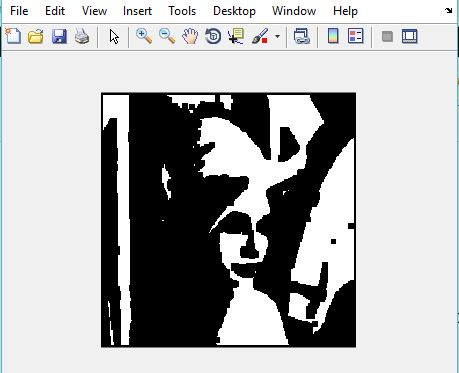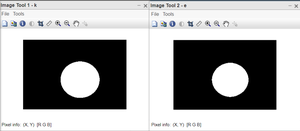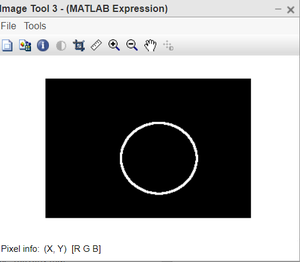# Matlab | Erosion of an Image

• Difficulty Level : Medium
• Last Updated : 30 Nov, 2021

Morphology is known as the broad set of image processing operations that process images based on shapes. It is also known as a tool used for extracting image components that are useful in the representation and description of region shape.

The basic morphological operations are:

• Erosion
• Dilation

Erosion:

• Erosion shrink-ens the image pixels i.e. it is used for shrinking of element A by using element B.
• Erosion removes pixels on object boundaries.:
• The value of the output pixel is the minimum value of all the pixels in the neighborhood. A pixel is set to 0 if any of the neighboring pixels have the value 0.

Approach:

• Using function im2bw(), convert the RGB image to a binary image.
• Create a structuring element or you can use any predefined mask eg. special(‘sobel’).
• Store the number of rows and columns in an array and loop through it.
• Create a zero matrix of the size same as the size of our image.
• Leaving the boundary pixels start moving the structuring element on the image and start comparing the pixel with the pixels present in the neighborhood.
• If the value of the neighborhood pixel is 0, then change the value of that pixel to 0.

Example:

## MATLAB

 `% Matlab code for Erosion``% read image``I=imread(``'lenna.png'``);  ` `% convert to binary ``I=im2bw(I);` `% create structuring element             ``se=ones(5, 5);` `% store number of rows``% in P and number of columns in Q.           ``[P, Q]=size(se);` `% create a zero matrix of size I.       ``In=zeros(size(I, 1), size(I, 2));` `for` `i=ceil(P/2):size(I, 1)-floor(P/2)``    ``for` `j=ceil(Q/2):size(I, 2)-floor(Q/2)` `        ``% take all the neighbourhoods.``        ``on=I(i-floor(P/2):i+floor(P/2), j-floor(Q/2):j+floor(Q/2));` `        ``% take logical se``        ``nh=on(logical(se));``      ` `        ``% compare and take minimum value of the neighbor``        ``% and set the pixel value to that minimum value.``        ``In(i, j)=min(nh(:));     ``    ``end``end` `imshow(In);`

Output:figure: Input imagefigure: Output Image

Let’s take another image to perform Erosion and here we use different MATLAB functions.

Syntax:

• strel() function is used to define the structuring element. We have chosen disk-shaped SE, of radius 5.
• imerode() function is used to perform the erosion operation.
• imtool() function is used to display the image.

Example:

## Matlab

 `% MATLAB code for Erison``% read the image.``k=imread(``"erosion.png"``);` `%define the structuring element.``SE=strel(``'disk'``,5);` `%apply the erosion operation.``e=imerode(k,SE);` `%display all the images.``imtool(k,[]);``imtool(e,[]);` `%see the effective reduction in org,image``imtool(k-e,[]);`

Output:Figure: Left: Original image, Right: Eroded imageFigure: Output image

Code explanation: